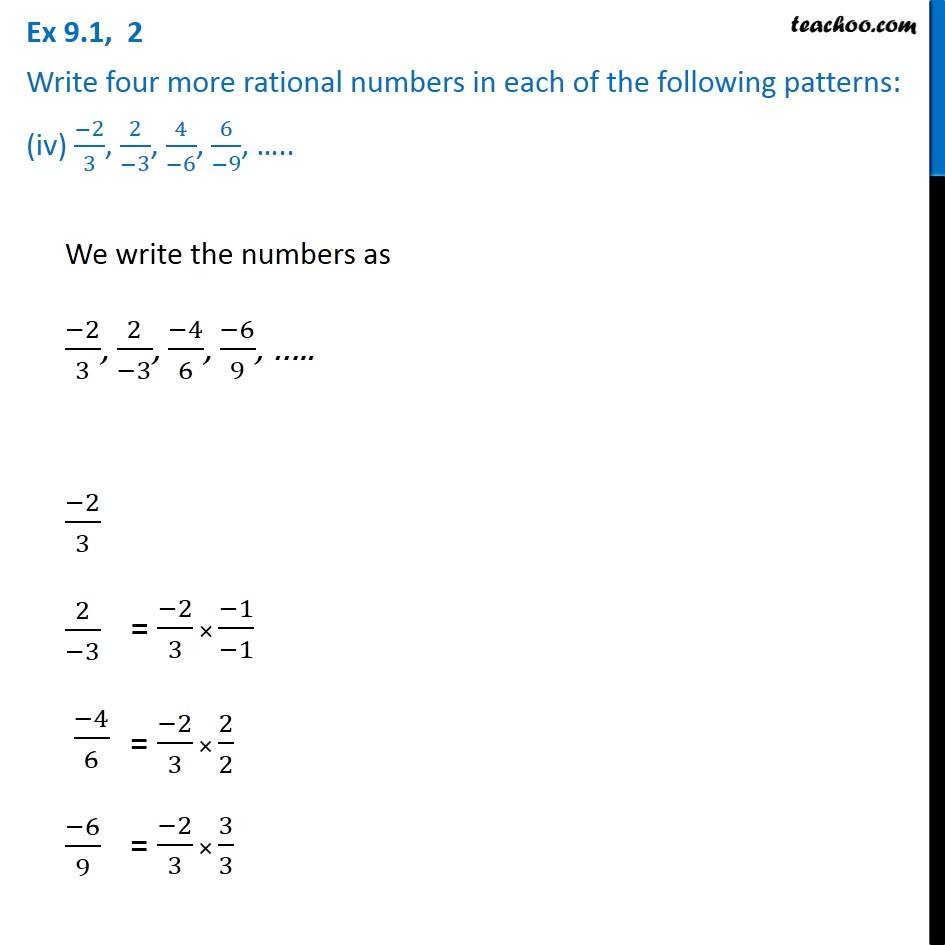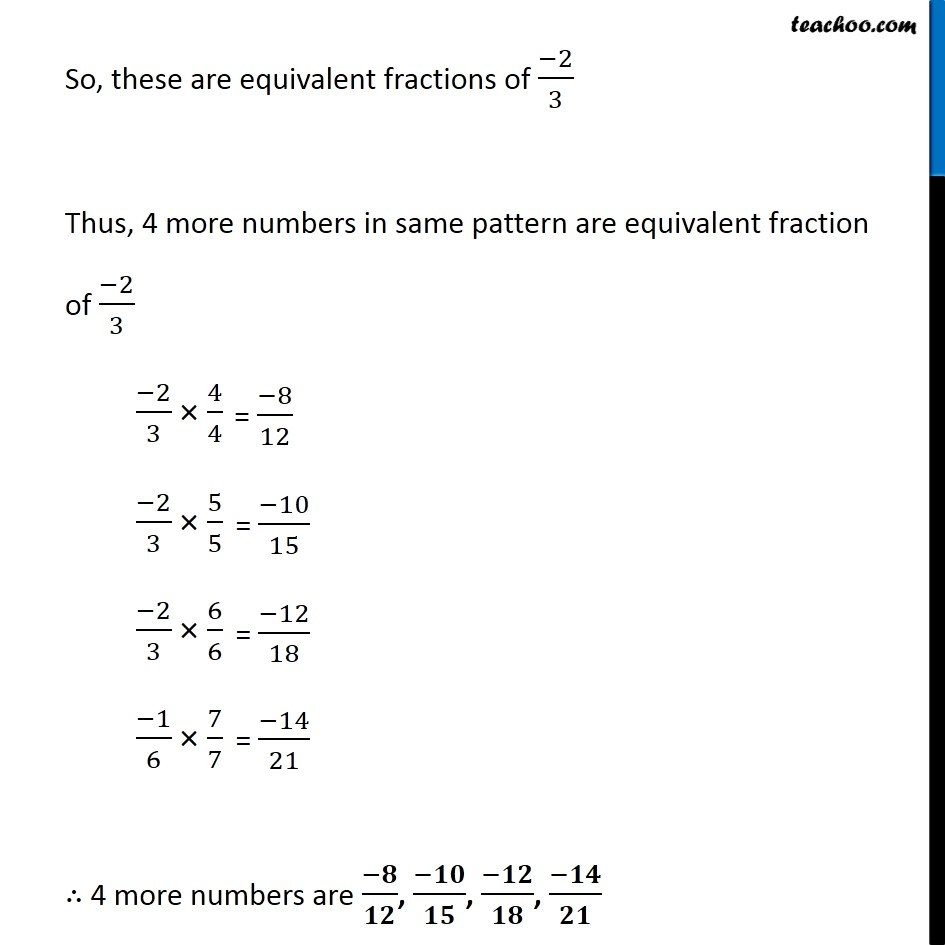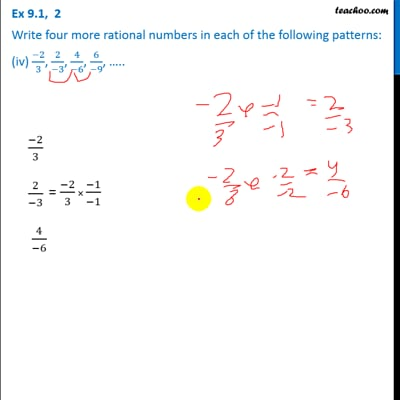Ex 9.1

Chapter 9 Class 7 Rational Numbers
Serial order wiseThis video is only available for Teachoo black users

Get live Maths 1-on-1 Classs - Class 6 to 12

### Transcript

Ex 9.1, 2 Write four more rational numbers in each of the following patterns: (iv) (−2)/3, 2/(−3), 4/(−6), 6/(−9), ….. We write the numbers as (−2)/3, 2/(−3), (−4)/6, (−6)/9, ….. (−2)/3 2/(−3) (−4)/6 (−6)/9 So, these are equivalent fractions of (−2)/3 Thus, 4 more numbers in same pattern are equivalent fraction of (−2)/3 (−2)/3 × 4/4 (−2)/3 × 5/5 (−2)/3 × 6/6 (−1)/6 × 7/7 ∴ 4 more numbers are (−𝟖)/𝟏𝟐, (−𝟏𝟎)/𝟏𝟓, (−𝟏𝟐)/𝟏𝟖, (−𝟏𝟒)/𝟐𝟏